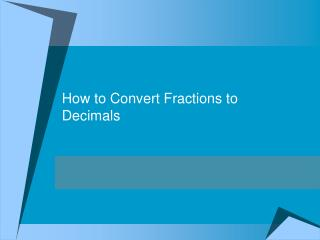DownloadDownload PresentationHow to Convert Fractions to Decimals

# How to Convert Fractions to Decimals

Download Presentation## How to Convert Fractions to Decimals

- - - - - - - - - - - - - - - - - - - - - - - - - - - E N D - - - - - - - - - - - - - - - - - - - - - - - - - - -
##### Presentation Transcript

1. How to Convert Fractions to Decimals

2. Objective:G5.1M.C1.PO1 I can convert fractions to decimals using division.

3. Essential Questions • 1. What are benchmark fractions, decimals, and percents? How are they related to each other? • 2. What are benchmark fractions, decimals, and percents? How would you compare them to part of • a whole? • 3. What are benchmark fractions, decimals, and percents? How can they be represented? • 4. What are the procedural steps to convert between fractions, decimals, and percents? Why are these procedures important? Why do we need to convert between fractions, decimals, and percents

4. How do we convert fractions into decimals? • We can use division. • Here are the steps

5. 1/2 2 1 First put the denominator on the OUTSIDE of the division house. Next, you put the numerator INSIDE the house. Think……NIDO!!!! Numerator Inside Denominator Outside!

6. Confusing??? Nah!!! . 2 1.0 Now just add a decimal and a 0 to the numerator. Then, line the decimal at the top. Like this!! Now, pretend the decimal is not even there! Easy as pie!!!!

7. Divide as normal! Remember DMSBR Divide, Multiply, Subtract, Bring Down, Repeat The difference…….NO REMAINDERS!

8. What decimal is ½??? . 2 1.0 Lets finish!!

9. Practice Time!! Please take out your slates! Change 2/3 into a decimal. Remember NIDO!!!

10. Let’s try another! Change 3/8 into a decimal.

11. Independent Work: Change the fractions to decimals 5/6 4/10 3/5 2/7

12. Closure: What is the procedure for turning a fraction into a decimal? Write it on your slate.